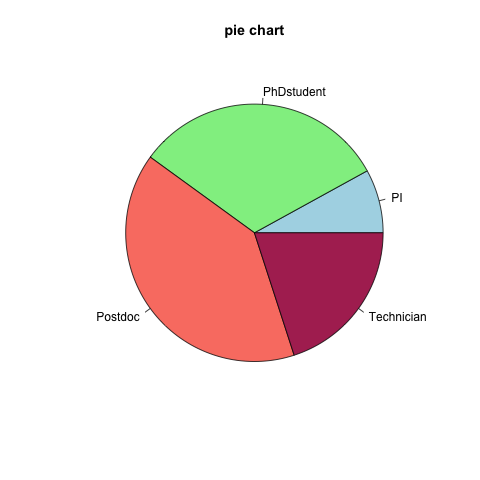## 16.3 Pie charts

A pie chart is a circular charts which is divided into slices, illustrating proportions.

• Using our previous vector, build a simple pie chart:
``````# Create a vector
x <- rep(c("PhDstudent", "Postdoc", "Technician", "PI"), c(8,10,5,2))

# Count number of occurences of each string
mytable <- table(x)

pie(mytable,
main="pie chart",
col=c("lightblue", "lightgreen", "salmon", "maroon"))``````